##### Trigonometry Workbook For DummiesSome trigonometry equations contain more than one trig function. Others have mixtures of multiple angles and single angles with the same variable. Some examples of such equations include 3cos2 x = sin2 x, 2sec x = tan x + cot x, cos 2x + cos x + 1 = 0, and sin x cos x = 1/2.

To get these equations into more-manageable forms so that you can use factoring or another method to solve them, you use identities to substitute for some or all of the terms. For example, to solve 3cos2 x = sin2 x for all the angles between 0 and 2π, apply a Pythagorean identity.

1. Replace the term sin2 x with its equivalent from the Pythagorean identity, sin2 x + cos2 x = 1 or sin2 x = 1 – cos2 x.

3cos2 x = 1 – cos2 x

2. Add cos2 x to each side and simplify by dividing.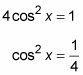3. Take the square root of each side.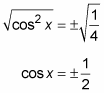4. Solve for the values of x that satisfy the equation.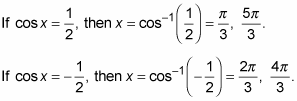In this next example, you begin with three different trig functions. A good tactic is to replace each function by using either a ratio identity or a reciprocal identity. Using these identities creates fractions, and fractions require common denominators.

By the way, having fractions in trig equations is good, because the products that result from multiplying and making equivalent fractions are usually parts of identities that you can then substitute in to make the expression much simpler. Solve 2sec x = tan x + cot x for all the possible solutions in degrees.

1. Replace each term with its respective reciprocal or ratio identity.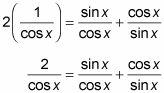2. Rewrite the fractions with the common denominator sin x cos x.

Multiply each term by a fraction that equals 1, with either sine or cosine in both the numerator and denominator.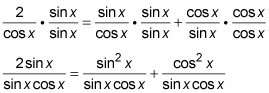3. Add the two fractions on the right. Then, using the Pythagorean identity, replace the new numerator with 1.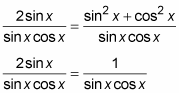4. Set the equation equal to 0 by subtracting the right term from each side.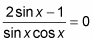5. Now set the numerator equal to 0.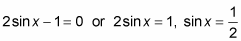If the numerator is equal to 0, then the whole fraction is equal to 0. The denominator shouldn’t be allowed to equal 0 — such a number doesn’t exist.

6. Solve for the values of x that satisfy the original equation.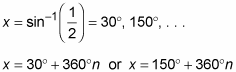In the next example, two different angles are in play. One angle is twice the size of the other, so you use a double-angle identity to reduce the terms to functions of only one angle. The trick is to choose the correct version of the cosine double-angle identity.

Solve cos 2x + cos x + 1 = 0 for x between 0 and 2p.

1. Replace cos 2x with 2cos2 x – 1.

2cos2x – 1 + cos x + 1 = 0

This version of the cosine double-angle identity is preferable because the other trig function in the equation already has a cosine in it.

2. Simplify the equation. Then factor out cos x.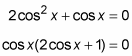3. Set each factor equal to 0.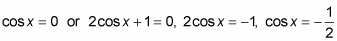4. Solve for the values of x that satisfy the original equation.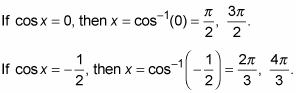This last example can be deceptively simple. The catch is that you have to recognize a double-angle identity upfront and make a quick switch.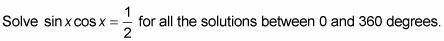1. Use the sine double-angle identity to create a substitution for the expression on the left.

Starting out with the identity and multiplying each side by 1/2, you get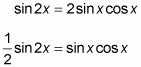2. Replace the expression on the left of the original equation with its equivalent from the double-angle identity.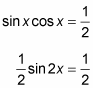3. Multiply each side of the equation by 2.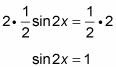4. Rewrite the expression as an inverse function.

2x = sin–1(1)

5. Determine which angles within two rotations satisfy the expression.

2x = sin–1(1) = 90°, 450°

You use two rotations because the coefficient of x is 2.

6. Divide each term by 2.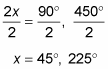Notice that the resulting angles are between 0 and 360 degrees.

You can generalize the double-angle technique from the above example for other multiple-angle expressions.